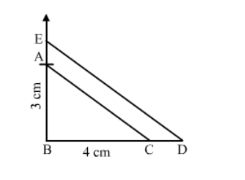# Construct a triangle with sides 5 cm, 6 cm and 7 cm and then another triangle whose sides are`
Question:

Construct a triangle with sides $5 \mathrm{~cm}, 6 \mathrm{~cm}$ and $7 \mathrm{~cm}$ and then another triangle whose sides are $\frac{7}{5}$ of the corresponding sides of first triangle.

Solution:

Steps of Construction :

Step 1. Draw a line segment BC = 4 cm.
Step 2. With B as centre, draw an angle of 90o.
Step 3. With B as centre and radius equal to 3 cm, cut an arc at the right angle and name it A.
Step 4. Join AB and AC.
Thus, △ ABC is obtained .

Step 5. Extend $B C$ to $D$, such that $B D=\frac{7}{5} B C=\frac{7}{5}(4) \mathrm{cm}=5.6 \mathrm{~cm}$.

Step 6. Draw DE ∥ CA, cutting AB produced to E.Thus, $\triangle E B D$ is the required triangle, each of whose sides is $\frac{7}{5}$ the corresponding sides of $\triangle A B C$.